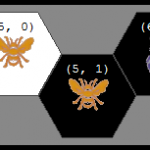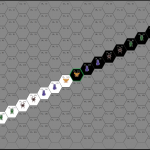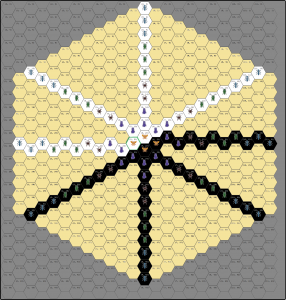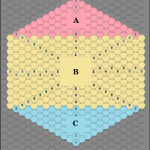# Visually debugging a Minimax AI engine

While creating the Steam version of the popular board game Hive, it became clear that normal methods of on-screen debugging weren’t going to be in-depth enough.

Most AI for 2-player abstract-strategy games probably uses the tried-and-true Minimax algorithm. It works just how you’d think AI would work: you look at a tree of all of the possible board positions that you could get to (and all positions you get to from there, etc.) and score them. It can get a little more complex on top of that, but the basics are really straightforward.

While the Minimax algorithm is very general-purpose, the scoring function that you use to evaluate each position is game-specific. Debugging that scoring function (called a “heuristic evaluation function” in technical lingo) can be tricky, especially since it is very hard to see an entire game-tree at once. The small tree in the first picture above is used for teaching minimax, but is unrealistically small for real games. As an example, even a very simple game like Tic-Tac-Toe would have 3 possible moves right away (it would be 9 moves, but the board is symmetrical, so there are only 3 actual different moves). Looking at this tic-tac-toe tree, you can see that even this extremely-simple game’s tree grows quite large by the third layer.

### Complexity of real Game TreesFirst two plies of Tic-Tac-Toe game tree

Let’s put that in perspective compared to other games: The number of moves that can be done per level is called a “branching factor“. In the tic-tac-toe example, the branching factor at the start of the game is 3. After moving, the branching factor is 2, 5, or 5, depending on which move is made. In chess, your first move can be one of 20 possibilities: 8 pawns (each with 2 different moves) and 2 knights which have 2 possible moves each. From there, the possibilities change very quickly. Due to this variability, when discussing games we tend to focus mainly on the average branching factor. The average branching factor of tic-tac-toe is 4, for chess it’s 35, the value for Hive is currently unknown but I’d estimate it between 40 and 50.

### The need for a good visualizer

For a game such as Hive, to have the AI look 3 levels deep, you’d have (50^3) = 125,000 nodes on the third level of the tree. Even if we limit the branching factor (which we do in our AI) the number of nodes is quite large. At the time of this writing one of our AI levels limits to a branching factor of 30. So (30^3) = 27,000 nodes. Needless to say, that tree won’t fit on most computer screens, so it would be hard to debug it all at once.

Therefore, we need a different way to visualize what’s going on. When debugging a heuristic evaluation function, it’s important to know the score throughout the tree, how the score worked its way up each level, and it also helps to be able to see a detailed view of how the AI scored the nodes. Keep in mind that only the scores on the bottom level of the tree are actually used to be the final scores of the path to that node. However, when we limit the branching-factor, that involves giving a preliminary score to each node and sorting all of the sibling nodes in any given level of the tree, before traversing to their children. This way, even though many moves may be skipped in a given level of the tree, the odds are high that the most important moves are being evaluated. Due to this pre-scoring, it is helpful to have detailed scoring information on every node in the tree.

In addition to seeing the nodes in a current level, it would be helpful to have pointers to which layers of the tree are maximization or minimization steps so that you don’t have to keep as much information in your head. This way, you can examine any node in the tree and tell where it got its score from (eg: it’s children) and how its score is being used by its parent-node if it has one.

### Our solution

We wanted to be able to run the AI in our game’s debug-mode, then create a log-file and view it easily. After looking around, it seemed that a very simple solution would be to dump some JSON in a format that the Javascript InfoVis Toolkit (JIT) could load, then create a tool to render a “Space Tree” which expands and collapses as needed.

→→ Check out our Minimax AI Visualizer Tool in action. ←←

I started with the SpaceTree demo from JIT and just modified it from there. Features:

• Loads data from a JSON file by default, but you can paste new JSON into a text-box to create a new tree (or use the text-box modify the existing tree).
• Colorized to easily show which steps are Maximize steps or Minimize steps
• Each node says what ply it represents to get to that node, and the final score that node ended up with.
• The mouse scroll-wheel lets you zoom in and out
• Hovering over a node brings up a detailed tool-tip bubble with the breakdown of each of the scoring-factors that were used to come up with the pre-scoring for a node – or the actual scoring, in the event that it’s a leaf-node.
• Hovering over the Root Node brings up a tool-tip bubble with detailed info on the entire run of the AI: how many nodes were evaluated, how long the AI ran, how many total prune events there were, etc..
• Each time there is a prune event (from the alpha-beta pruning), there will be one node which indicates the entire number of sibling nodes that were pruned at once.

If you want to use the same tool to visualize the progress of your own AI, all you need to do is have your code output JSON in the same format that’s used in the textarea below the graph, then paste it into that textarea and hit the “Load Tree” button.

### Outcome

Being able to more quickly track-down some of the weird decisions that the AI was making, let us drastically improve the AI in only a few days of work. The example that’s embedded in the Visualizer is from before most of the changes, but that shouldn’t matter since it’s just showing how it works. We’ve had some very good players helping us debug it, and one of the recent World Champions said that the top level of AI made him force a draw. We’re getting there!

If you want to see the AI in action, check out Hive on Steam.

If you have any questions about the Visualizer or about our AI, let me know in the comments!

# Calculating the bounding board for Hive

THIS POST IS LONG! If you don’t care to see how the answers were calculated and just want to know the size of the 3D area into which all possible Hive games could fit, just skip to the bottom of the post for the results.

While doing secondary-research to get ready for writing AI for our Xbox version of Hive, I noticed that there wasn’t much published about the game-theory for Hive yet. That’s strange for such a popular abstract-strategy game but the game, but I guess someone has to be the first …so it’s going to be us!

This is hopefully the first post in a series which will seek to start more in-depth research on Hive by calculating some of the common measures of the complexity of Hive.

This post is going to show how we calculated the bounding-board for Hive. For a very easy comparison, chess and checkers are played on a square board that has 8 rows and 8 columns and thus has 64 spaces. Since Hive doesn’t have a static board, and instead is created by whatever undirected graph of hexagonal tiles is laid down by the players, calculating the bounding board is less straightforward.

### Hive Board Size Calculation – Background

Quick background:

• As shown in the official Hive rules, the basic game has 11 black tiles and 11 white tiles for a total of 22 tiles. Our calculations will refer to this core version of the game without modifications unless noted otherwise.
• For the sake of calculations and nomenclature, we’ll be using the coordinate system described in our recent post about representing hexagonal boards in 2-dimensional arrays. This post will be very hard to follow if you haven’t read that.
• Since the Hive board can be reshaped dynamically and could actually be moved end-over-end in any direction indefinitely, there is no actual physical board that you could make which could be used to contain an ongoing game. Rather, the “bounding board” we seek to calculate is the smallest area in which absolutely every legal permutation of board states could be contained. In more simple English: we could use this to make a box such that any legal game of Hive could be covered with this box. If we lift the box and another play is made, we could again cover the board with the box (even if the box is in a slightly different location).

### Step 1: Building the bounding board

Now it’s time to start imagining all of the extremes. To make the examples easier to visualize, we will picture all of the white tiles on one side and all of the black tiles on the other side, with their point of contact being the queen from each side. Now we can build the board:

1. Because of the way our coordinate system works, if all of the tiles were layed out from (0,0) downward so that the next piece was at (0,2), then all of tiles would take up 44 rows and 1 column. This is the most extreme vertical case.
2.If the tiles were laid out to maximize the number of columns, they would follow a zig-zag pattern from the left to the right. Since each two pieces would equal one-column, then there would be 5 columns taken up by white pieces, then the white queen and black queen would share the center column, followed by 5 more columns of black tiles. This leads to 11 columns. This can be done in 2 rows, but the next example will show that the number of rows used could also be increased.

3.To create the most extreme diagonals, interestingly, the number of columns does not decrease at all in this coordinate system. However, when the pieces are laid out so that they are all 45-degrees off from each other, the maximum number of rows now increases to 22.

4.If all of the extreme examples are superimposed upon each other, the minimum bounding-area becomes clear. Awesomely enough, the bounding area is a heaxagon! Patterns everywhere, man. It tickles my math-parts that the hexagon is also rotated 90-degrees from the orientation of the hexagonal tiles themselves.

### Step 2: Measuring the bounding board

Since the board is not a simple square, a bit more math needs to be done in order to calculate the size of the bounding area. This will be done for the general-case of Hive in terms of the number of tiles which each color has available. This gives the bonus of making it trivial to calculate a maximum board-size for the other existing variants to Hive also.

It’s actually pretty easy to calculate the size of the bounding hexagon:

 The number of tiles which each color has will be called “X”. In the default Hive game, for example, there are 11 tiles of each color. eg: `X = 11` For simplicity, the hexagon can be broken into three parts. Triangle A on top, rectangle B in the middle, and triangle C on the bottom.The number of spaces (the area) of the bounding board is just the sum of these parts. `Area = A + B + C` This is a hexagon, so A is just a mirror-image of C, but the size of A and C is the same `A = C`, so `Area = 2A + B` The way hexagons get laid out in a triangular shape makes it so that row 1 has 1 tile, row 2 has 2 tiles, etc. Therefore, the area of A is the triangle number of X. We’ll use T(X) to represent the triangle number of X. `A = T(X)` B is a rectangle on the hexagonal grid, so it contains rows whose width fluctuates between being X tiles wide and X-1 tiles wide. Therefore, we can say that B is the sum of the longer rows (which appear on the top and bottom) plus the sum of the narrower rows (of which there is one-fewer because they are neither on the top of the rectangle nor on the bottom). `Area = 2T(X) + B``B = (sum of pieces in the wider rows) + (sum of pieces in the narrower rows)` The wider rows are X+1 tiles wide, and the whole rectangle is X tiles high. `sum of pieces in the wider rows = ((X+1 tiles) * X rows)` The narrower rows are X tiles wide and there is one less row of these because they are bounded on each side by the wider rows. `sum of pieces in the narrower rows = (X tiles * (X-1) rows)` Therefore… `B = ((X+1) * X) + (X * (X-1))` Substituting that back into the area equation we get… `Area = 2T(X) + ( ((X+1) * X) + (X * (X-1)) )`

So that gives us the maximum area of the bottom layer of the minimum bounding board of Hive. Bottom layer, you say? Yup: we haven’t accounted for that wily Beetle yet! For the unacquainted: the Beetle is a special piece in Hive which has the ability to climb on top of other tiles and trap them.

### Step 2.5: Modifying the total to take height into account – a.k.a. “What about the Beetle!?”

Since Hive pieces can be stacked, the board is technically 3D: it is a hexagonal prism. Therefore we need the total volume of the board to measure the number of board-spaces.

In Game Theory, it’s most important to create an upper-bound on the number of board spaces (cells), the state-space complexity (number of valid board configurations), and game-tree complexity (all possible moves), rather than necessarily having the exact number. While the calculations shown above for the bottom-layer of the board are exact, when taking height into account we can over-estimate a little (for simplicity) to get a general case equation for the upper-bound of all variants of Hive.

Since the total number of spaces (on all levels) needs to be calculated, the equation can be modified by multiplying the maximum size of a layer by the maximum height the board can achieve. The maximum height that the tiles can achieve will be referred to as H, so the new equation is:

`Max board spaces = H * (2T(X) + ( ((X+1) * X) + (X * (X-1)) ))`

### Step 3: Applying the equation: the actual upper-bound of the Hive board size!

As mentioned above, this is a slight over-counting which we might explain and tweak in a later post, but this will still give us an important start if we just fill in the numbers to the general-case equation above.

Plug and chug:

 General equation derived above `Max board spaces = H * (2T(X) + ( ((X+1) * X) + (X * (X-1)) ))` Hive without any modifications has 11 tiles of each color. `X = 11` In the basic edition of Hive, the maximum height is achieved by piling all 4 Beetles (2 from each color) on top of the same non-Beetle tile. This maximum height, therefore is 5. H = 5 The general equation for Triangle numbers is `T(X) = (X * ((X+1)/2))` `T(11) = (11 * ((11+1)/2))``T(11) = 66` Substitute these values in `Max board spaces = 5 * ((2*66) + ( ((11+1) * 11) + (11 * (11-1)) ))` The maximum possible number of board-spaces in the smallest bounding region which could contain any legal configuration of a game of Hive with no modifications: `Max board spaces in Hive = 1,870`

So there you have it, there are no more than 1,870 spaces in the virtual board for Hive. For comparison, chess (which is pretty complex already) has 64 spaces.

Did you have as much fun with that as I did? 😀

Please comment if you’ve looked over my reasoning and it seems solid, if you’ve found some errors to correct, or if you have any questions.
Thanks!

Update: In the comments, Hive Champion, Randy Ingersoll, helps narrow it down to 1,290 spaces in the virtual board for Hive.